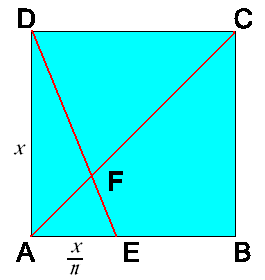#### You may also like### Triangle Midpoints

You are only given the three midpoints of the sides of a triangle. How can you construct the original triangle?### Pareq Exists

Prove that, given any three parallel lines, an equilateral triangle always exists with one vertex on each of the three lines.### The Medieval Octagon

Medieval stonemasons used a method to construct octagons using ruler and compasses... Is the octagon regular? Proof please.

# Folding Fractions

##### Age 14 to 16Challenge Level

Richard of Mearns Castle High School sent us in this solution:Notice that $AE = \frac{1}{n} AB = \frac{1}{n}x$

We can say that $\triangle AEF$ and $\triangle DCF$ are similar. This is because $\angle AFE$ is equal to $\angle DFC$ (vertically opposite). Also $\angle FAE = \angle FCD$ and $\angle AEF = \angle CDF$ (alternate angles).

Since the triangles are similar we can say that the ratios of corresponding sides are the same. Therefore:
\eqalign{ \frac {DC}{AE} &= \frac{FC}{AF}\cr \frac{x}{\frac{x}{n}}&= \frac{FC}{AF}\cr n &= \frac{FC}{AF} }
Hence$$FC = AF \times n$$
So $DE$ cuts $AC$ at the ratio $1:n$.